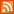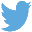Bucaro TecHelpHTTPS Encryption not required because no account numbers orpersonal information is ever requested or accepted by this siteCustom Search# Java Operators

Operators are special characters used to manipulate operands. Operators that perform an operation on one operand are called unary. Operators that perform an operation on two operands are called binary. Operators that perform an operation on three operands are called ternary.

Arithmetic Operators

If variables a and b are integer types and a holds 8 and b holds 4:

 Operator Name Description Example + addition Adds values a + b result is 12 - subtraction Subtracts value a - b result is 4 * multiplication Multiplies values a * b result is 32 / division Divides value a / b result is 2 % modulus Divides value returns remainder a % b result is 0 -- decrement Decreases value by 1 a-- result is 7 ++ increment Increases value by 1 a++ result is 9

Relational Operators

If variables a and b are integer types and a holds 8 and b holds 4:

 Operator Name Description Example == equal to if values are equal result is true, if not equal resut is false a == b is false != not equal to if values are not equal result is true, if values are equal result is false a != b is true > greater than if value of left operand is greater than value of right operand, result is true, if not resut is false a > b is true < less than if value of left operand is less than value of right operand, result is true, if not resut is false a < b is false >= greater than or equal to if value of left operand is greater than or equal to value of right operand, result is true, if not resut is false a >= b is true <= less than or equal to if value of left operand is less than or equal to the value of right operand, result is true, if not resut is false a <= b is false

Bitwise Operators

Bitwise operators work on bits and performs bit-by-bit operation. Bitwise operators can be applied to the integer, long, int, short, char, and byte variable types.

If variable a = 0011 1100 binary and variable b = 0000 1101 binary:

 Operator Name Description Example & bitwise AND binary AND a & b result is 0000 1100 | bitwise OR binary OR a | b result is 0011 1101 ^ bitwise XOR binary XOR a ^ b result is 0011 0001 ~ bitwise compliment binary ones complement ~a result is 1100 0011 << left shift binary shift left a << 2 result is 1111 0000 >> right shift binary shift right a >> 2 result is 1111 1111 >>> shift right zero fill shift right zero fill a >>> 2 result is 0000 1111

When you shift right (>>), the leftmost bits exposed by the shift are filled in with the previous contents of the leftmost bit This serves to preserve the sign of negative numbers when you shift right and is called sign extension. That's why we need shift right zero fill, in case you whast the the leftmost bits filled with zeros.

Logical Operators

If variable a holds true and variable b holds false:

 Operator Name Description Example && logical AND if both operands are non-zero, the condition becomes true a && b is false || logical OR if any of the two operands are non-zero, the condition becomes true a || b is true ! logical NOT if condition is true, result is false. If condition is false result is true !(a && b) is true

 .menubtn { margin-top:10px; margin-left:50px; width:220px; opacity:1; border-style:solid; border-color:#009000; background-color:#66ff66; transition-property: opacity; transition-duration: 1s; transition-timing-function: ease-out; } .menubtn:hover { opacity:1; background-color:#e5ff23; } Menu - More Java Programming MenuRSS FeedFollow @Stephen Bucaro Java[Site User Agreement] [Privacy Policy] [Site map] [Search This Site] [Contact Form]
Copyright©2001-2023 Bucaro TecHelp 13771 N Fountain Hills Blvd Suite 114-248 Fountain Hills, AZ 85268